Tuesday 21st September 2021

CBSE Guess > Papers > Question Papers > Class XII > 2006 > Physics > Physics Compartment Outside Delhi Set - I.

Physics — 2006 (Set I — Compartment Outside Delhi)

Q. 1. Write two properties of a material used as a suspension wire in a moving coil glvanometer. 1

Q. 2. Name the experiment which establishes the wave nature of a particle. 1

Q. 3. Write the S.I. unit for the activity of a radoactive nuclide. 1

Q. 4. The a.c. current gain of a transistor is 120. What is the change in the collector current in the transistor whose base current changes by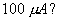1

Q. 5. Name the underlying principle of the working of an optical fibre. 1

Q. 6. Define ‘dielectric constant’ of a medium. Briefly explain why the capacitance of a parallel plate capacitor increases, oii introducing a dielectric medium between the plates. 2

Q. 7. Why does a paramagnetic substance display greater magnetisation for the same magnetising field when cooled? How does a diamagnetic substance respond to similar temperature changes? 2

Q. 8. Explain how electron mobility changes for a good conductor when (i) the temperature of the conductor is decreased at constant potential difference and (ii) applied potential difference is doubled at constant temperature. 2

Q. 9. A capacitor and a resistor are connected in series with an a.c. source. If the potential difference across C, R are 120 V, 90 V rspectively and if the r.m.s. current of the circuit is 3 A, calculate the (i) impedance, (ii) power factor of the circuit. 2

Or

An inductor 200 mH, a capacitor C and a resistor 10 ohm are connected in series with a 100 V, 50 s-1 a.c. source. If the current and voltage arc in phase with each other, calculate the capacitance of the capacitor.

Q. 10. Draw the curve showing variation of binding energy/nucleon with mass number of different nuclei. Briefly state, how nuclear fusion and nuclear fission can be explained on the basis of this graph. 2

Q. 11. Give r for the following:

(a) Rainbow is never observed on the surface of the Moon.
(b) The Sun looks reddish at sunrise and sunset as viewed from Earth.

Q. 12. The following figure shows the input waveforms (A, B) and the output waveform (Y) of a gate. Identify the gate and write its truth table. 2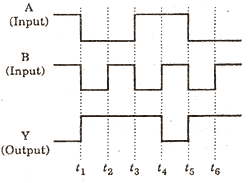Q. 13. What are eddy currents? How are these minimised? Mention two applications of eddy currents. 3

Q. 14. Draw the graphs showing the variation of photoelectric current with an ode potential of a photocell for (i) the same frequencies but different intensities I1 > I2 > I3, of incident radiation, (ii) the same intensity but different frequencies V1 > V2 > V3 of incident radiation.
Explain why the saturation current is independent of the anode potential. 3

Q. 15. State Faraday’s laws of electrolysis. Deduce the relation connecting Faraday constant, chemical equivalent and electrochemical equivalent. 3

Q. 16. 4 cells of identical emf E, internal resistance r, are connected in series to a variable resistor. The following graph shows the variation of terminal voltage of the combination with the current output: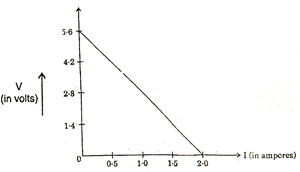(i) What is the emf of each cell used?
(ii) For what current from the cells, does maximum power dissipation occur in the circuit?
(iii) Calculate the internal resistance of each cell. 3

Q. 17. What is pulse modulation? Distinguish between pulse amplitude modulation and pulse duration modulation by drawing suitable modulated signals. 3

Q. 18. Write any two criteria required for a detector of an optical communication system.
A photo-detector is made from a semiconductor having Eg = 0.73 eV. What is the maximum wavelength which it can detect? 3

Q. 19. Using Gauss’ theorem, deduce an expression for the electric field intensity at any point due to a thin, infinitely long wire of charge/length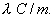3

Or

Using Gauss’ theorem, derive an expression for the electric field intensity at any point outside a charged spherical shell of radius R and of charge density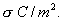Q. 20. A metallic square loop ABCD of size 15 cm and resistance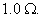is moved at a uniform velocity of v m/s, in a uniform magnetic field of 2 Tesla, the field lines being normal to the plane of the paper. The loop is connected to an electrical network of resistors, each of resistance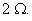Calculate the speed of the loop, for which 2 m A current flows in the loop.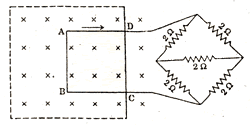Q. 21. Sketch a graph showing the variation of potential energy of a pair of nucleons as a function of their separation.
Write three characteristic properties of nuclear force which distinguish it from the electrostatic force. 3

Q. 22. Explain, using Huygen’ principle, how diffraction is produced by a narrow slit which is illuminated by a monochromatic light.
Show that central maximum is twice as wide as the other maxima and the pattern becomes narrower as the width of the slit is increased. 3

Q. 23. Draw a labelled diagram o the experimental set-up used by Hertz to produce electromagnetic waves. Explain how the electromagnetic waves are detected. 3

Q. 24. Define the term electric potential due to a point charge.
Calculate the electric potential at the centre of a square of side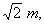having charges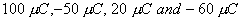at the four corners of the square. 3

Q. 25. Derive an expression for the magnetic field along the axis of an air-cored solenoid, using Ampere’s circuital law.
Sketch the magnetic field lines for a finite solenoid. Explain why the field at the exterior mid-point is weak while at the interior it is uniform and strong. 5

Or

Explain with the help of a labelled diagram the working principle of a cyclotron.
Show that the cyclotron frequency does not depend on the speed of the particle. Write one of the uses and point out one of the drawbacks of a cyclotron.

Q. 26. Draw a labelled circuit diagram of a transistor oscillator. Briefly explain its principle and state how the oscillations are sustained. 5

Or

Draw the energy band diagrams of p-type, n-type semiconductors. Explain with a circuit diagram the working of a full wave rectifier.

Q. 27. (i) Draw a labelled ray diagram of a compound microscope, showing the formation of image at the near point of the eye.
(ii) A compound microscope uses an objective lens of focal length 4 cm and eye lens of focal length 10cm. An object is placed at 6 cm from the objective lens.
(a) Calculate magnifying power of the compound microscope, if the final image is formed at the near point.
(b) Calculate the length of the compound microscope also. 5

Or

Draw a labelled ray diagram of an astronomical telescope, forming the image at infinity.
As astronomical telescope uses two lenses of powers 10 diopter, 1 diopter.
(i) State with reason, which lens is preferred as objective and eye-piece.
(ii) Calculate the magnifying power of the telescope, if the final image is formed at the near point.
(iii) How do the light gathering power and resolving power of a telescope change, if the aperture of the objective lens is doubled ?

 Physics 2006 Question Papers Class XII Delhi Outside Delhi Compartment Delhi Compartment Outside DelhiSet ISet ISet ISet I

 CBSE 2006 Question Papers Class XIIEnglishSociologyFunctional EnglishPsychologyMathematicsPhilosopyPhysicsComputer ScienceChemistryEntrepreneurshipBiologyInformatics PracticesGeographyMultimedia & Web TechnologyEconomicsBiotechnologyBusiness StudiesPhysical EducationAccountancyFine ArtsPolitical ScienceHistoryAgriculture